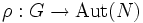# Finite-characteristic-semidirectly extensible implies linearly pushforwardable over prime field

This fact is related to: Extensible automorphisms problem
View other facts related to Extensible automorphisms problemView terms related to Extensible automorphisms problem |

## Statement

Suppose$G$ is a finite group and$p$ is a prime not dividing the order of$G$. Let$k$ be the prime field with$p$ elements. Then, any finite-characteristic-semidirectly extensible automorphism of$G$ is linearly pushforwardable over$k$.

## Definitions used

### Finite-characteristic-semidirectly extensible automorphism

Further information: finite-characteristic-semidirectly extensible automorphism

An automorphism$\sigma$ of$G$ is termed finite-characteristic-semidirectly extensible if, for any homomorphism$\rho:G \to \operatorname{Aut}(N)$ where$N$ is a finite group, such that$N$ is a characteristic subgroup in the semidirect product$M = N \rtimes G$, the automorphism$\sigma$ extends to an automorphism of$M$.

### Linearly pushforwardable automorphism

Further information: Linearly pushforwardable automorphism An automorphism$\sigma$ is termed linearly pushforwardable for a group$G$ over a field$k$ if, for any finite-dimensional linear representation$\rho:G \to GL(V)$, there exists$a \in GL(V)$ such that for every$g \in G$, we have:$\rho(\sigma(g)) = a\rho(g)a^{-1}$